Barry Davies
sunago@internet.co.nz

### To Double a Cube -- The Solution of Archytas

Here follows Heath's presentation:

"After Hippocrates had discovered that the duplication of the cube was equivalent to finding two mean proportionals in continued proportion between two given straight lines, the problem seems to have been attacked in the latter form exclusively. [. . .]

Archytas

The solution of Archytus is the most remarkable of all, especially when his date is considered (first half of fourth century B. C.), because it is not a construction in a plane but a bold construction in three dimensions, determining a certain point as the intersection of three surfaces of revolution, (1) a right cone, (2) a cylinder, (3) a tore or anchor-ring with inner diameter nil. The intersection of the two later surfaces gives (says Archytas) a certain curve (which is in fact a curve of double curvature), and the point required is found as the point in which the cone meets this curve.

Suppose that AC, AB are the two straight lines between which two mean proportionals are to be found, and let AC be made the diameter of a circle and AB a chord in it.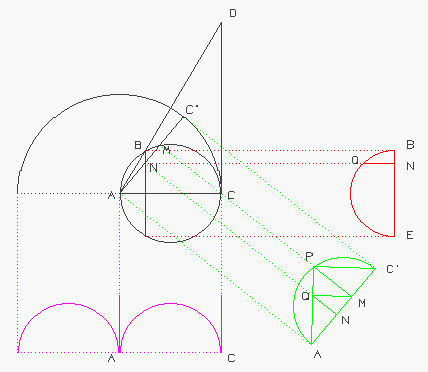Draw a semicircle with AC as diameter, but in a plane at right angles to the plane of the circle ABC, and imagine this semicircle to revolve about a straight line through A perpendicular to the plane of ABC (thus describing half a tore with inner diameter nil).

Next draw a right half-cylinder on the semicircle ABC as base; this will cut the surface of the half-tore in a certain curve.

Lastly let CD, the tangent to the circle ABC at the point C, meet AB produced in D; and suppose the triangle ADC to revolve about AC as axis. This will generate the surface of a right circular cone; the point B will describe a semicircle BQE at right angles to the plane of ABC and having its diameter BE at right angles to AC; and the surface of the cone will meet in some point P the curve which is the intersection of the half-cylinder and the half-tore.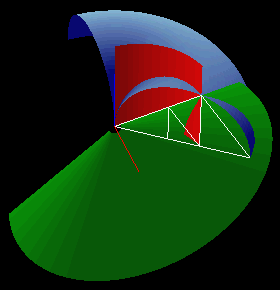[The white triangle is APC'.]

Let APC' be the corresponding position of the revolving semicircle, and let AC' meet the circumference ABC in M.

Drawing PM perpendicular to the plane of ABC, we see that it must meet the circumference of the circle ABC because P is on the cylinder which stands on ABC as base.

Let AP meet the circumference of the semicircle BQE in Q, and let AC' meet its diameter in N. Join PC', QM, QN.

Then, since both semicircles are perpendicular to the plane ABC, so is their line of intersection QN (Eucl.XI. 19).

Therefore QN is perpendicular to BE.

Therefore QN.QN = BN.NE = AN.NM, (Eucl. III. 35) so that the angle AQM is a right angle.

But the angle APC' is also right; therefore MQ is parallel to C'P.

It follows, by similar triangles, that

C'A:AP = AP:AM = AM:AQ;
that is
AC:AP = AP:AM = AM:AB,

and AB, AM, AP, AC are in continued proportion, so that AM, AP are the two mean proportionals required.

In the language of analytical geometry, if AC is the axis of x, a line through A perpendicular to AC in the plane of ABC the axis of y, and a line through A parallel to PM the axis of z, then P is determined as the intersection of the surfaces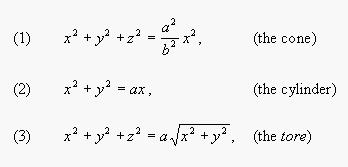where AC=a, AB=b.

From the first two equations we obtain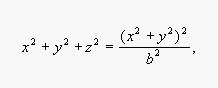and from this and (3) we have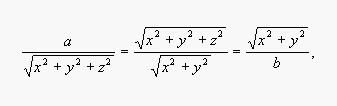or
AC:AP = AP:AM = AM:AB.

Compounding the ratios, we have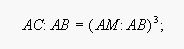therefore the cube of side AM is to the cube of side AB as AC is to AB.

In the particular case where AC = 2 AB,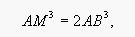and the cube is doubled."

Quoted with permission from: A History of Greek Mathematics by Sir Thomas Heath; vol 1, pp 246-249; Dover Publications, Inc. 31 East 2nd Street, Mineola NY 11505: This Dover edition, first published in 1981, is an unabridged republication of the work first published in 1921 by the Clarendon Press, Oxford. For this edition the errata of the first edition have been corrected.